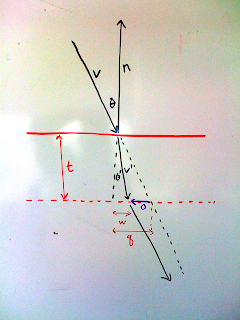## Thursday, October 9, 2008

### Double-sided dielectrics for ray tracingNo, neither I nor this blog is dead yet.

Sometimes ray tracing models will take a thin dielectric (like a car windshield) and represent it with one surface. If we assume this dielectric surface is locally planer, then the direction of the ray will not be changed by the double refraction, but it will be offset. This is shown in the image above. Our challenge is to calculate the vector o in the figure. The new origin of the ray will just be hit_point+o.

The thickness t is provided by the model, possibly with a texture. First let's find a unit vector parallel to o by subtracting off the portion of v in the direction of n:

a = -normalize(v - dot(v,n)n)

Now we need to find the distance that is the length of o, which is q-w. We can see that

q = t tan(Theta).

Similarly,

w = t tan(Theta')

Snell's law tells us that

sin(Theta) = ref_Idx sin(Theta').

Assuming v is a unit vector we also have

cos(Theta) = -dot(v,n)

That allows us (with a bunch of sqrts) to get all the sines and cosines we need above.